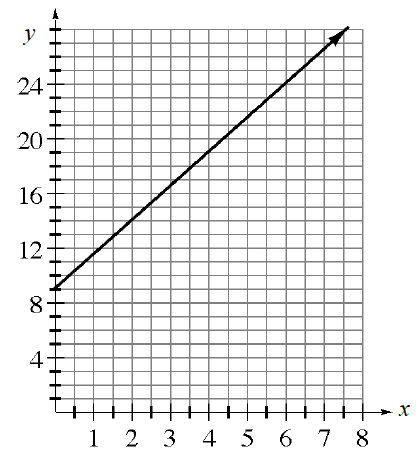### Home > CCA2 > Chapter A > Lesson A.3.1 > ProblemA-92

A-92.

Use the given information to find an equation of the line.

1. Slope $2$ and passing through $(10, 17)$.

Use the Slope-Intercept Form for the equation of a line.
Substitute $2$ for $m$, and $(10, 17)$ for $x$ and $y$.

$y = 2x - 3$

2. Passing through $(1, -4)$ and $(-2, 5)$.

Find the slope, then use the Slope-Intercept for the equation of a line.

3.  x y $-6$ $-3$ $0$ $3$ $6$ $-6$ $-4$ $-2$ $0$ $2$

Find the slope and $y$-intercept.

$y=\frac{2}{3}x-2$

1.Find the slope and $y$-intercept.